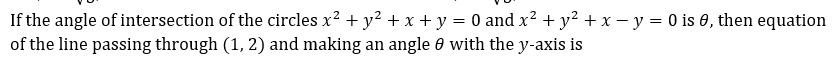## [LATEST]\$type=sticky\$show=home\$rm=0\$va=0\$count=4\$va=0

In JEE exams, Conic section is one of the most important topic of Mathematics that comes under Coordinate Geometry, it has total of 15 percent weightage out of which 6% questions are asked in JEE mains and 9% in Advance.

•  {-1,1}
•  {-2,2}
•  {-2,1/2}
•  {2,-1/2}
Q3. Let C be a circle with two diameters intersecting at an angle of 30°. A circle S is tangent to both the diameters and to C, and has radius unity. The largest radius of C is
•  1+√6+√2
•  1+√6-√2
•  √6+√2-11
•  None of these
•  2√3
•  4√3
•  3√2
•  2√5
Q5. Two circles with radii a and b touch each other externally such that θ is the angle between the direct common tangents (a>b≥2), then
Q6. In triangle ABC, equation of side BC is x-y=0. Circumcentre and orthocenter of the triangle are (2, 3) and (5, 8), respectively. Equation of circumcircle of the triangle is
••••• Solution
Q7.Q8.•  (1+√2,-2)
•   (1-√2,-2)
•  (1,-2+√2)
•  None of these
Solution
Q9. On the line segment joining (1, 0) and (3, 0), an equilateral triangle is drawn having its vertex in the fourth quadrant, then radical centre of the circles described on its sides as diameter is
•  (3,-1/√3)
•  (3,-√3)
•  (2,-1/√3)
•  (2,-√3)
Q10.•  x=1
•  y=2
•  x+y=3
•  x-y=3
Solution#### Written by: AUTHORNAME

AUTHORDESCRIPTION## Want to know more

Please fill in the details below:

Name